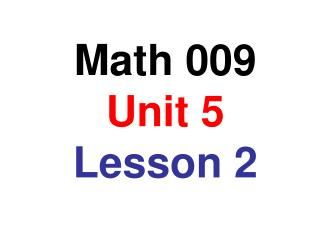DownloadDownload PresentationMath 009 Unit 5 Lesson 2

# Math 009 Unit 5 Lesson 2

Télécharger la présentation## Math 009 Unit 5 Lesson 2

- - - - - - - - - - - - - - - - - - - - - - - - - - - E N D - - - - - - - - - - - - - - - - - - - - - - - - - - -
##### Presentation Transcript

1. Math 009 Unit 5Lesson 2

2. Constants, Variables and Terms A variable is represented by a letter x is a variable A number is often called a constant -9 is a constant A term is a variable or a constant or the product of a constant and variables. x is a term 7 is a term 12x is a term -4x3 is a term The terms of an expression are separated by addition signs or subtraction signs The expression x2 – 3x + 5 has three terms

3. Identifying the parts of a term 5x3 x is the variable of the term 5 is the coefficient of the term 3 is the exponent of the variable What is the coefficient of the term: -17x7 What is the exponent of the term: 23x10

4. Identifying like terms Two terms are called like terms if their variable parts are exactly the same – including any exponents. • 5x and -3x • x2 and x • and -3 • 11x2 and 5x2 • 3x and 3 like terms not like terms like terms like terms not like terms

5. Distributive Property If a, b, and c are real numbers, then a(b + c) = ab + ac The Distributive Property can also be written this way ab + ac = (b + c)a This second form helps in combining like terms Simplify the following: 5x + 3x = (5 + 3)x 5y2 – 11 y2 = (5 – 11)y2 = 8x = - 6y2 Terms cannot be combined by addition or subtraction unless they are like terms. Can you simplify this process, so that you don’t have to write so much each time you do a problem?

6. The process of combining like terms can be summarized as • add or subtract the coefficients of like terms as indicated • the variable part remains unchanged Simplify each of the following: 2x + 3y – 7x + 10y 8x – 15x + 7 2x – 7x + 3y + 10y -7x + 7 -5x + 13y -3x2 + 7 + 8x2 – 14 + x – 5x 8x2 – 3x2 + x – 5x + 7 – 14 5x2 – 4x – 7

7. Simplifying expressions by combining like terms Simplify each of the following 8x – 3 + 5x + 18 = 8x + 5x – 3 + 18 = 13x + 15 9x + 5y – 3x – 2y = 9x – 3x + 5y – 2y = 6x + 3y 6u + 7v – 8 – 12v + 14 + 9u = 6u + 9u + 7v – 12v – 8 + 14 = 15u – 5v + 6

8. Simplifying expressions by combining like terms Simplify each of the following 3x2 – 2x + 5 – x2 – 18 = 3x2 – x2 – 2x + 5 – 18 = 2x2 – 2x – 13 -7x2 + 4xy + 8x2 – 12xy = -7x2 + 8x2 + 4xy – 12xy = x2 – 8xy 7x2y – 15xy2 + 3 + 12xy2 – 8x2y = 7x2y – 8x2y – 15xy2 + 12xy2 + 3 = -x2y – 3xy2 + 3

9. Simplifying expressions involving the Distributive Property Remember that multiplications are done before additions or subtractions 6y + 2(2y + 3) = 6y + 4y + 6 = 10y + 6 7(2x – 5) + 3(4x – 3) = 14x – 35 + 12x – 9 = 14x + 12x – 35 – 9 = 26x – 44

10. Simplifying expressions involving the Distributive Property Remember that multiplications are done before additions or subtractions -3(2a – 5) – 2(4a + 3) = -6a + 15 – 8a – 6 = -6a – 8a + 15 – 6 = -14a + 9 2c – 3(c + 4) – 2(2c – 3) = 2c – 3c – 12 – 4c + 6 = 2c – 3c – 4c – 12 + 6 = - 5c – 6